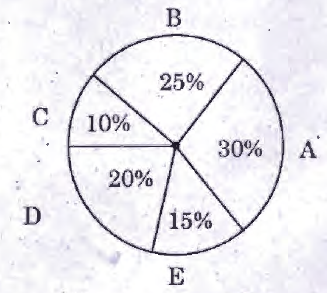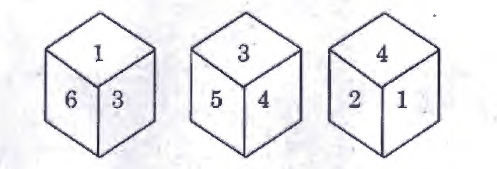# Group 4 2013 August GE TNPSC Question Paper

## Group 4 2013 August GE TNPSC Questions

21.
Who was the first woman judge of the supreme Court?
A.
Hanna Chandy
B.
Vijayalakshmi Pandit
C.
Indira Gandhi
D.
Fathima Beevi
D. Fathima Beevi
22.

Consider the following statements :

Assertion (A) : The (ARC) recommended that the institution of Lokpal and Lokayakta.

Reason (R) : 1. should be demonstratively Independent and Impartial.

2. their appointment should be as far as possible non - political.

A.

Both (A) and (R) are false

B.

Both (A) and (R) are correct

C.

(A) is false but (R) is true

D.

(A) is true but (R) is false

B. Both (A) and (R) are correct

23.

Arrange the President of India in chronological order of their term in Office.

I. R. Venkatraman

II. Dr. Sankar Dayal Sharma

III. Dr. K.R. Narayanan

IV. Dr. A.P.J. Abdul Kalam

A.

I, II, III, IV

B.

III, IV, I, II

C.

III, I, II, IV

D.

III, II, I, IV

A. I, II, III, IV

24.
The Bhopal Gas Tragedy happened in
A.
1980
B.
1978
C.
1975
D.
1984
D. 1984
25.
The books 'Wings of Fire' was written by
A.
Nehru
B.
A.P.J. Abdul Kalam
C.
Kamaraj
D.
Mahatma Gandhi
B. A.P.J. Abdul Kalam
26.
In the products 2a x 1a = 41a; 2b x 1b = 37b, a and b represent positive integers. Then a + b =
A.
15
B.
11
C.
7
D.
13
B. 11
27.
Find the odd man out in the following : 1, 144, 16, 25, 49, 81, 121, 36, 62
A.
1
B.
49
C.
121
D.
62
D. 62
28.

The World-Wide Tea product of % countries for the year 2011-2012.The angle subtended by E at the centre of the circle is

A.

15⁰

B.

30⁰

C.

54⁰

D.

72⁰

C. 54⁰

29.
In an examination 30% of total students failed in English, 40% of students failed in Hindi and 20% in both. Find the percentage of students who passed in both the subjects.
A.
50%
B.
20%
C.
10%
D.
60%
A. 50%
30.

Three positions of the same die are given. Find the number opposite to the side represented by 2.A.

5

B.

3

C.

6

D.

1